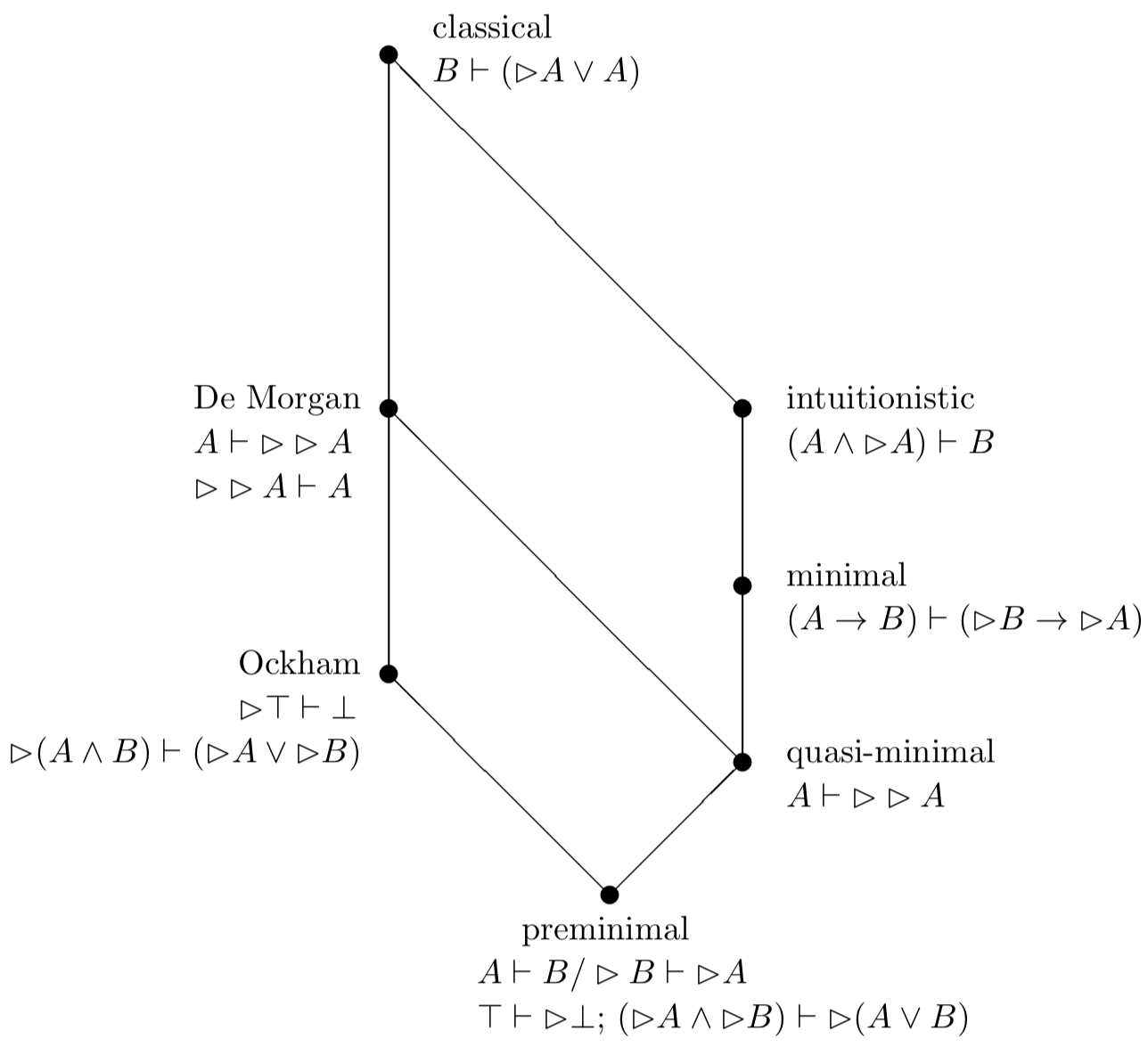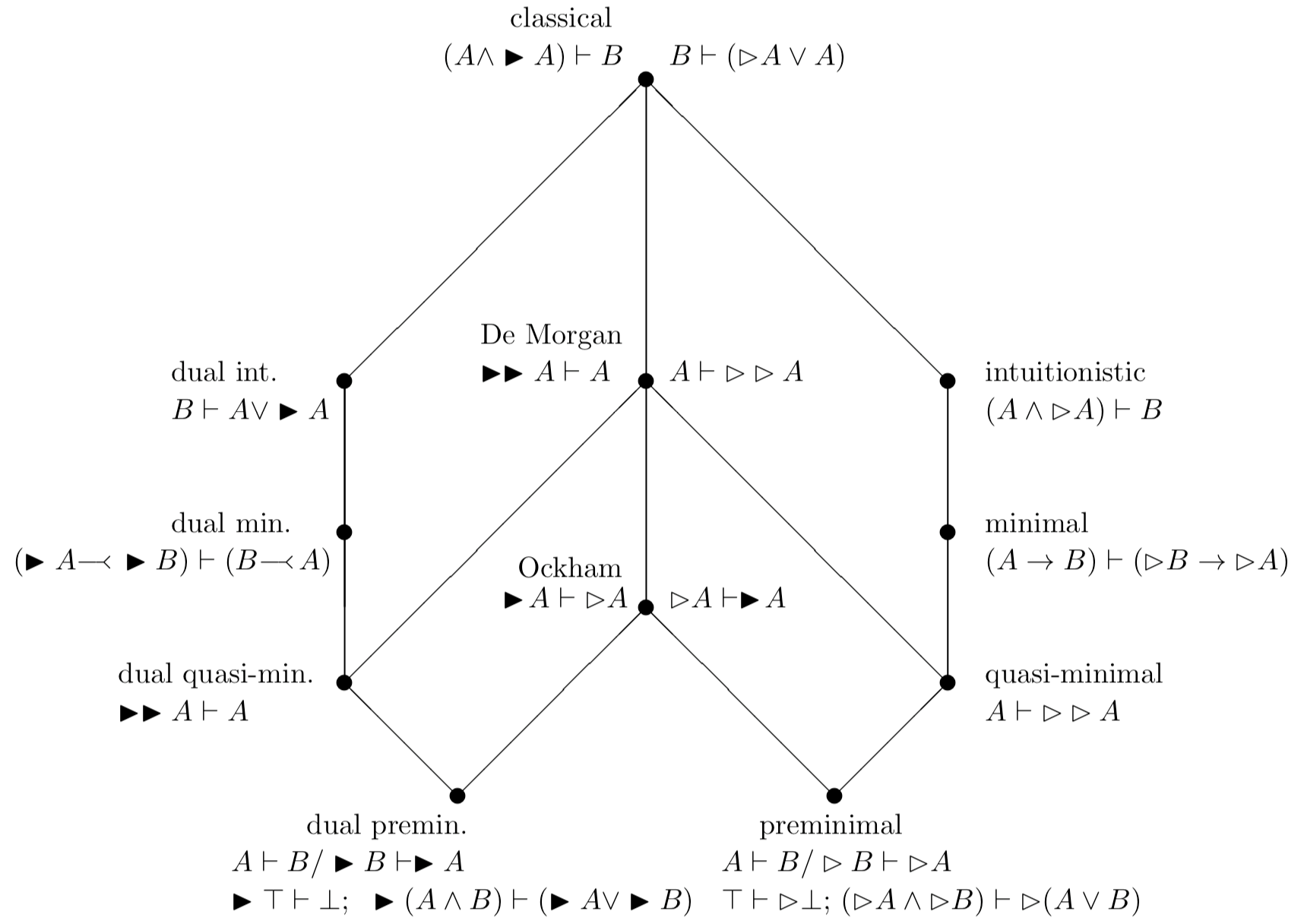## Substructural negations: negation as a modal operator in display calculi

In sections 2.3 and 2.3, negation as a modal operator in increasingly rich vocabularies has been considered primarily in the setting of formula-to-formula sequents, with pointers to work by Lahav, Marcos, and Zohar, who use sequents with finite sets of formulas on the left and the right hand side of the turnstile, $$\vdash$$, and work by Colacito, de Jongh, and Vargas, who make use of Hilbert-style axiom systems. This section presents negation still as a normal modal operator, but now using a generalization of Gentzen's sequent calculus, namely display calculi. In this setting, properties of negation are captured by sequent rules that do not exhibit any operations from the logical object language but only connectives from the structural language of sequents. The negations under consideration can be obtained from classical negation by giving up structural sequent rules and can therefore be called “substructural negations.” Display calculi were introduced by Nuel Belnap (1982), and the modal display calculus was introduced in Wansing 1994 (see also Wansing 1998, 2002). In the modal display calculus, there is a unary structure connective, $$\bullet$$, which has a context-sensitive intuitive reading. On the left of the turnstile it is to be understood as the backward-looking possibility operator from temporal logic, P (“sometimes in the past”), and on the right of the turnstile, it is to be read as the forward-looking necessity operator, G (“always in the future”). The operators P and G form a residuated pair, which means that $$A \vdash$$G$$B$$ is valid just in case P$$A \vdash$$$$B$$ is valid. If $$X, Y$$ are structures, then the basic structural rules governing $$\bullet$$, its display rules, are:

$X \vdash \bullet Y \, / \, \bullet X \vdash Y \mbox{ and }\bullet X \vdash Y \, / \, X \vdash \bullet Y.$

The framework of substructural negations has been developed by Onishi (2015), starting from bi-intuitionistic logic. Onishi introduces four negations as normal modal operators, namely forward-looking impossibility, $$\rhd$$, and backward-looking impossibility, $$\lhd$$, as well as forward-looking unnecessity, $$\blacktriangleright$$, and backward-looking unnecessity, $$\blacktriangleleft$$. Both pairs come with their own binary accessibility relation, namely $$\frown$$ as the compatibility relation used to interpret negation as impossibility, and $$\smile$$ as a separate relation of “exhaustiveness” used to interpret negation as unnecessity.

Onishis's BiN-frames are then compatibility frames with an additional binary exhaustiveness relation: $$(W, \frown, \smile, \leq)$$ or, in Onishi's presentation, $$(W, \leq, \frown, \smile)$$. (Onishi does not require the relation $$\leq$$ to be anti-symmetric, which makes no difference concerning the set of valid formulas.) A BiN-model adds to a BiN-frame a persistent valuation function, and suitable conditions on the relations $$\leq$$, $$\frown$$, and $$\smile$$ are imposed so as to ensure that persistence holds for arbitrary formulas. The verification conditions for negated formulas are then as already explained:

$\begin{array}{l} M, w \models \rhd A \mbox{ iff } \mbox{for all } u \in W\!: w\frown u \mbox{ implies } M, u \not \models A, \\ M, w \models \lhd A \mbox{ iff } \mbox{for all } u \in W\!: u\frown w \mbox{ implies } M, u \not \models A, \\ M, w \models \blacktriangleright A \mbox{ iff } \mbox{there exists } u \in W\!: w\smile u \mbox{ and } M, u \not \models A, \\ M, w \models \blacktriangleleft A \mbox{ iff } \mbox{there exists } u \in W\!: u\smile w \mbox{ and } M, u \not \models A. \\ \end{array}$

The structural language of display sequents makes use of various context-sensitive operations: a structural constant, $$\mathbf{I}$$, two unary operations, $$\sharp$$ and $$\flat$$, and two binary structure connectives, ; and >. Every formula is a structure, and the structure operations are used to build up more complex structures. Structures appear in antecedent and succedent position of a sequent.

Two slightly different display calculi for bi-intuitionistic logic have been presented in Goré 2000 and Wansing 2008. Onishi uses a variant of the sequent rules from Goré 2000. The structure connectives have a context-dependent reading depending on whether they appear in antecedent or succedent position of a sequent. Their intuitive understanding as operations from the logical object language is as follows:

$\begin{array}{rccccccc} & \mathbf{I} & ; & > & \sharp & \flat & \\ \mbox{in antecedent position} & \top & \wedge & \coimp & \blacktriangleright & \blacktriangleleft \\ \mbox{in succedent position} & \bot & \vee & \rightarrow & \rhd & \lhd \\ \end{array}$

This understanding justifies the following display rules for $$\sharp$$ and $$\flat$$ and the following left and right introduction rules for the four negation connectives:

$$\sharp X \vdash Y \, / \, \flat Y \vdash X$$ and $$\flat Y \vdash X \, / \, \sharp X \vdash Y$$,

$$X \vdash \sharp Y \, / \, Y \vdash \flat X$$ and $$Y \vdash \flat X \, / \, X \vdash \sharp Y$$,

$$X \vdash A \, / \, \rhd A \vdash \sharp X$$, $$\quad X \vdash \sharp A \, / \, X \vdash \rhd A$$, $$\quad X \vdash A \, / \, \lhd A \vdash \flat X$$, $$\quad X \vdash \flat A \, / \, X \vdash \lhd A$$,

$$\sharp A \vdash X \, / \, \blacktriangleright A \vdash X$$, $$\quad A \vdash X \, / \, \sharp X \vdash \blacktriangleright A$$, $$\quad \flat A \vdash X \, / \, \blacktriangleleft A \vdash X$$, $$\quad A \vdash X \, / \, \flat X \vdash \blacktriangleleft A$$.

If these rules are added to the display calculus for bi-intuitionistic logic, one obtains a sequent calculus $$\delta$$BiN that admits cut-elimination. The following sequent rules and sequents are valid on any BiN-frame (the first rule is admissible, the remaining rules and sequents are derivable in $$\delta$$BiN):

$$A \vdash \rhd B \, / \, B \vdash \lhd A,$$ $$A \vdash B \, / \, \rhd B \vdash \rhd A,$$ $$B \vdash \lhd A \, / \, A \vdash \rhd B,$$ $$\top \vdash \rhd \bot,$$ $$\rhd A \wedge \rhd B) \vdash \rhd (A \vee B).$$

The following list of sequents are valid on a BiN-frame just in case the first-order condition given above it is satisfied (the corresponding structural sequent rule is given on the right):

• $$\forall x \forall y (x \frown y \Rightarrow y \frown x)$$
$$A \vdash \rhd \rhd A \qquad X \vdash \sharp Y \, / \, Y \vdash \sharp X$$
• $$\forall x \forall y (x\frown y \Rightarrow \exists z ( x \leq z \amp z \leq y \amp x \frown z))$$:
$$(A \rightarrow B) \vdash (\rhd B \rightarrow \rhd A) \qquad X \vdash \sharp (Y ;Z) \, / \, X \vdash Y > \sharp Z$$
• $$\forall x \, x\frown x$$
$$(A \wedge \rhd A) \vdash B \qquad X \vdash \sharp Y \, / \, X \vdash Y > \mathbf{I}$$
• $$\forall x \exists y \, x \frown y$$
$$\rhd \top \vdash \bot \qquad X \vdash \sharp \mathbf{I} \, /\, X \vdash \mathbf{I}$$
• $$\forall x \forall y (x \frown y \Rightarrow y \leq x)$$
$$B \vdash A \vee \rhd A \qquad (X; Y) \vdash Z \, / \, X \vdash (\sharp Y ; Z)$$
• $$\forall x \forall y \forall z ((x \frown y \amp x \frown z) \Rightarrow \exists w (y \leq w \amp z \leq w \amp x \frown w))$$
$$\rhd (A \wedge B) \vdash (\rhd A \vee \rhd B) \qquad X \vdash \sharp (Y;Z) \, / \, X \vdash (\sharp Y ; \sharp Z)$$
• $$\forall x \exists y (x \frown y \amp \forall z (y \frown z \Rightarrow z \leq x))$$
$$\rhd \rhd A \vdash A$$

Whereas the first six of the above sequents correspond with the listed purely structural sequent rules, for double negation elimination it is not clear whether there exists a corresponding structural rule. From the point of view of proof-theoretic semantics (see the entry on proof-theoretic semantics) these correspondences are appealing for it may be held that the above introduction rules of the four negations once and for all fix their meaning, so that variations of meaning can be spelled out in purely structural terms. The emerging kite of negations as impossibility is shown in Figure 5.Figure 5

Onishi then defines a dualization function, $$(\cdot )^t$$ (notation adjusted), that maps formulas from the language with $$\rhd$$ and $$\lhd$$ into formulas from the language with $$\blacktriangleright$$ and $$\blacktriangleleft$$, and vice versa. In particular,

$\begin{array}{ccccccc} (A \wedge B )^t & = & (A ^t \vee B^t) & \; & (A \vee B )^t & = & (A ^t \wedge B^t) \\ (A \rightarrow B )^t & = & (B^t \coimp A^t) & \; & (A \coimp B )^t & = & (B ^t \rightarrow A^t) \\ (\rhd A)^t & = & \blacktriangleright A ^t & \; & (\lhd A )^t & = & \blacktriangleleft A ^t \\ (\blacktriangleright A)^t & = & \rhd A ^t & \; & (\blacktriangleleft A )^t & = & \lhd A ^t \\ \end{array}$

This translation is extended to sequents and to the first-order language of conditions on frames, so as to obtain dual frame correspondences for sequents in the language with $$\blacktriangleright$$ and a dual kite of negations as unnecessity, charting negations dual to the ones form the kite of negations as impossibility. Moreover, Onishi notes that the dual Ockham, dual de Morgan, and dual classical negations are just the Ockham, de Morgan, and classical negations expressed by unnecessity. Since the characteristic principles of preminimal negation as impossibility are translated as the characteristic principles of negation as unnecessity, and vice versa, the identification of $$\rhd$$ and $$\blacktriangleright$$ results in Ockham negation. The axioms that express the identification of negation as impossibility and negation as unnecessity, $$\rhd A \vdash \blacktriangleright A$$ and $$\blacktriangleright A \vdash \rhd A$$, correspond with the following frame conditions and structural sequent rules:

$\begin{array}{ccc} \rhd A \vdash \blacktriangleright A & \forall x \exists y (x \frown y \amp x \smile y) & \flat X \vdash \flat Y \, / \, Y \vdash X \\ \blacktriangleright A \vdash \rhd A & \forall x \forall y \forall z ((x \frown y \amp x \smile z) \Rightarrow y \leq z) & X \vdash Y \, / \, \sharp Y \vdash \sharp X \\ \end{array}$

With the identification of negation as impossibility and negation as unnecessity we are led to the united kite of negations shown in Figure 6.Figure 6

Note that in the presence of the axioms for identifying negation as impossibility and negation as unnecessity and their frame conditions, every negation from the united kite emerges as substructural because both double negation elimination for $$\blacktriangleright$$ and double negation introduction for $$\rhd$$ do correspond with a structural sequent rule.

Onishi also points out that although the logic of Ockham negation is complete with respect to frames that satisfy the Star Postulate (see the supplement document “Additional Conceptions of Negation as a Unary Connective”), the Star Postulate does not correspond with the combination of the sequents $$\rhd \top \vdash \bot$$ and $$\rhd (A \wedge B) \vdash (\rhd A \wedge \rhd B)$$ that give rise to the logic of Ockham negation when added to the basic display calculus $$\delta$$BiN. The two frame conditions corresponding with $$\rhd A \vdash \blacktriangleright A$$ and $$\blacktriangleright A \vdash \rhd A$$, however, are equivalent to the stronger condition that requires for every state $$w$$ the existence of a state $$w^*$$ that is not only maximal among the states compatible with $$w$$ but also minimal among the states that are jointly exhaustive with $$w$$, so that $$M, w \models \rhd A$$ iff $$M, w^* \not \models A$$ iff $$M, w \models \blacktriangleright A$$.